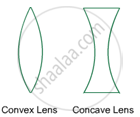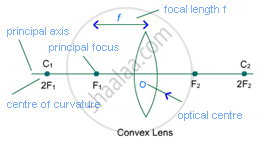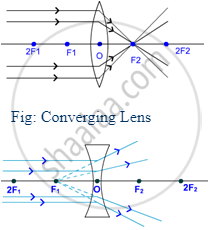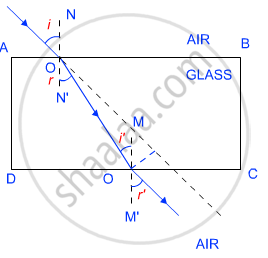# Refraction of Light

#### Topics

• Partial reflection and refraction at the boundary of two different media
• Cause of refraction
• Some consequences of refraction of light

## Notes

SPHERICAL LENS

Lens is an optical device which converges or diverges the rays of light before transmitting. A lens has similar shape to lentils and genus of lentil is called Lens, thus a lens got its name after the shape and name of genus of lentils. A lens is made by combining at least one part of sphere made of transparent material, generally glass.

Spherical Lens: Most of the lenses are made by the combination of parts of transparent sphere. Concave and Convex lens are most commonly use spherical lens.

Convex lens is the most commonly used lens in our day to day life.

Convex lens:

A lens having two spherical surface bulging outwards is called Convex Lens. It is also known as biconvex lens because of two spherical surface bulging outwards.### Concave lens:

A lens having two spherical surface bulging inwards is called Concave Lens. It is also known as biconcave lens because of two spherical surface bulging inwards.

#### Important terms for spherical lens:Centre of curvature: The centre of sphere of part of which a lens is formed is called the centre of curvature of the lens. Since concave and convex lenses are formed by the combination of two parts of spheres, therefore they have two centres of curvature.

One centre of curvature is usually denoted by C1 and second is denoted by C2.

Focus: Point at which parallel rays of light converge in a concave lens and parallel rays of light diverge from the point is called Focus or Principal Focus of the lens.Similar to centres of curvature; convex and concave lenses have two Foci. These are represented as F1 and F2.

Principal Axis: Imaginary line that passes through the centres of curvature of a lens is called Principal Focus.

Optical centre: The central point of a lens is called its Optical Centre. A ray passes through optical centre of a lens without any deviation.

Radius of curvature: The distance between optical centre and centre of curvature is called the radius of curvature, which is generally denoted by R.

Focal Length: The distance between optical centre and principal focus is called focal length of a lens. Focal length of a lens is half of the radius of curvature.

2F = R

Or, F = "R"/2

This is the cause that the centre of curvature is generally denoted by 2F for a lens instead of C.

## Notes

REFLECTION OF LIGHT

Reflection is one of the unique properties of light. It is the reflection of light, which enables us to see any object.

Reflection: The bouncing back of rays of light from a polished and shiny surface is called reflection or reflection of light. It is similar to bouncing back of a football after colliding with a wall or any hard surface.

Laws of reflection:-

(i) The angle of incidence is equal to the angle of reflection, and

(ii) The incident ray, the normal to the mirror at the point of incidence and the reflected ray, all lie in the same plane.

The angle of incidence is denoted by 'i' and angle of reflection is denoted by 'r'. The law of reflection is applicable to all types of reflecting surface.

Mirror is a shiny polished object (glass) which reflects most of the rays of light falling upon it. One side of mirror is polished with suitable material to make the other side reflective.

Real Image: Image which is formed in front of the mirror and it can be obtained on a screen is called real image.

Virtual Image: Image which is formed behind the mirror and cannot be obtained on a screen is called virtual image.

## Notes

REFRACTION:

The change of direction of light because of change of medium is known as Refraction or Refraction of Light. The ray of light changes its direction or phenomenon of refraction takes place because of difference in speed in different media.

The light travels at faster speed in rare medium and at slower speed in denser medium. The nature of media is taken as relative. For example air is a rarer medium than water or glass.

When ray of light enters from a rarer medium into a denser medium, it bends towards normal at the point of incidence. On the contrary, when ray of light enters into a rarer medium from a denser medium it bends away from the normal.

Ray emerging after the denser medium goes in the same direction and parallel to the incident ray.

The angle between incident ray and normal is called Angle of Incidence and it is denoted as ‘i’. The angle between refracted ray and normal is called the Angle of Refraction. Angle of refraction is denoted by ‘r’.Laws Of Refraction Of Light.

(i) The incident ray, the refracted ray and the normal to the interface of two transparent media at the point of incidence, all lie in the same plane.

(ii) The ratio of sine of angle of incidence to the sine of angle of refraction is a constant, for the light of a given colour and for the given pair of media. This law is also known as Snell’s law of refraction. (This is true for angle 0 < i < 90o) If i is the angle of incidence and r is the angle of refraction, then,

"sinI"/"sinr" = constant

This constant value is called the refractive index of the second medium with respect to the first.

If you would like to contribute notes or other learning material, please submit them using the button below.

#### Video Tutorials

We have provided more than 1 series of video tutorials for some topics to help you get a better understanding of the topic.

Series 1

Series 2

Series 3

### Shaalaa.com

Light Reflection and Refraction part 1 (Introduction) [00:05:07]
S
0%